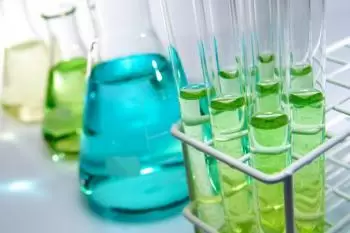# What Is Chemical Energy?

Chemical energy is the potential of a chemical to undergo transformation through a chemical reaction or to transform into other chemicals. Forming or breaking chemical bonds involves energy. This energy can be absorbed or evolved from a chemical system.The energy that can be released (or absorbed) by a reaction between a set of chemicals is equal to the difference between the amount of energy in the products and the reactants. This change in energy is called the internal energy of a chemical reaction.

Since the strength of chemical bonds is associated with the distance between chemical species (in fact the strongest chemical bonds are those that have the chemical elements involved in the closest bond), chemical energy depends on the mutual position of the particles that constitute a substance. Therefore, chemical energy is the energy stored in chemical bonds. This energy is largely attributable to the sum of the potential energy of the electrostatic interactions of the charges present in the ponderable matter, plus the kinetic energy of the electrons. This energy is also present at the junction of subatomic particles (protons and neutrons) in the nucleus of an atom. It is what is called nuclear energy.

If you have a "zero level" of chemical energy that is where there are no chemical bonds, the chemical energy is negative.

Resonance is a chemical-structural phenomenon that greatly influences chemical energy in the stabilizing sense. The reactivity and kinetics of the various compounds suffer from the differences in chemical energy.

Chemical energy is produced in the course of transformations that take place at the molecule level.

## Chemical Energy and Thermodynamics

Chemical energy is closely related to thermodynamics in which concepts of internal energy, enthalpy and thermodynamic processes appear.

In thermodynamic chemistry the term used for potential energy is chemical potential, and the Gibbs-Duhem equation is often used for chemical transformation.

The change in internal energy of a process is equal to the change in heat if measured under conditions of constant volume, as in a calorimeter. However under constant pressure conditions the measured heat is not always equal to the internal energy change. The change of heat at constant pressure is called the enthalpy change.

## Use of Chemical Energy in Technical Systems

Another useful term is the heat of combustion, which is the energy released in the combustion reaction and often applied to the study of fuels.

From a technical point of view, fuels contain chemical energy that is converted into mechanical energy through their combustion, for example, in thermal engines. The fuel allows the transformation of the chemical reaction energy from combustion directly into electrical energy or mechanical energy.

When batteries are used, chemical energy is directly converted into electrical energy through electrochemical redox reactions. An accumulator behaves similarly to a battery in energy use, and vice versa, can convert electrical energy into chemicals and store it.

## Potential Chemical Energy

Potential chemical energy is a form of potential energy related to the structural arrangement of atoms or molecules. This arrangement may be the result of chemical bonds between the molecules. The chemical energy of a substance can be transformed into other forms of energy by chemical reaction.

The similar term chemical potential is used to indicate the potential of a substance to undergo a configuration change, in the form of a chemical reaction, space transport, particle exchange with a reservoir.

### Examples of Chemical Energy

Here are a few examples of chemical energy:

• Nuclear energy. In nuclear fission or fusion reactions, chemical alterations are generated in the atoms that are transformed into chemical energy.
• Combustion of a fuel. An example of this case we have when burning a fuel and obtaining thermal energy in the form of heat.
• Digestion by a living organism. Food, through certain reactions in the stomach, is converted into chemical energy for the body.
• Photosynthesi. Green plants obtain energy by transforming solar energy into chemical energy in photosynthesis, electrical energy can be converted into chemical energy through electrochemical reactions.

Nuclear chemistry is a branch of chemistry that deals with the study of the chemical aspects mentioned in:

• nuclear properties (such as atomic nucleus structure, nuclear reactions, and radioactive decay)
• properties of the elements of the end of the periodic system (for which nuclear radiation detection is essential)
• macroscopic phenomena involving nuclear processes (for example, radiochronology, astrophysics, etc.)
• application of analysis techniques based on nuclear phenomena (such as activation analysis or the use of radioactive tracers) in the study of scientific problems in a variety of scientific or technical fields.

## Nuclear Chemistry

What is nuclear chemistry?

Nuclear chemistry is a branch of chemistry that deals with the study of the chemical aspects mentioned in:

• nuclear properties (such as atomic nucleus structure, nuclear reactions, and radioactive decay)
• properties of the elements of the end of the periodic system (for which nuclear radiation detection is essential)
• macroscopic phenomena involving nuclear processes (for example, radiochronology, astrophysics, etc.)
• application of analysis techniques based on nuclear phenomena (such as activation analysis or the use of radioactive tracers) in the study of scientific problems in a variety of scientific or technical fields.

Nuclear chemistry is closely linked to radiochemistry, which in turn deals with the study of natural and artificial radioactivity and the use of radioisotopes in the study of chemical processes. Radiochemistry includes activities such as radioanalytical methods, applications of radionuclides in disciplines outside the area of ​​chemistry (for example, nuclear medicine), radioisotopes of chemistry (in direct relation to physics), material chemistry, highly radioactive and isotopic labeling methods .

Author:

Published: December 11, 2015
Last review: February 17, 2020